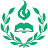System of two linear equations in two variables | Algebra I | Tutors Panel AcademySystem of two linear equations in two variables | Algebra I | Tutors Panel Academy

How to solve system of two linear equations involving two variables?

Watch the next lesson:

Missed the previous lesson?

Algebra 1 on Tutors Panel Academy: In Algebra 1 we will be discussing expressions, systems of equations, functions, real numbers, inequalities, exponents, polynomials, radical and rational expressions. We will also think about linear equations, functions, polynomials, factoring, and much more!

About Tutors Panel Academy: Tutors Panel Academy is an independent, personalized and convenient 1-On-1 online tutoring platform for the learners. We have taken one step ahead to enable practical thinking along with on-the-fly exercises to measure the students’ absorbing caliber. We are focused on taking students education and learning to the next level.

Our “3-in-Box” model has been setup to not only provide high quality critical thinking, but also to enhance student’s competitive skills and learn the essence of education. In short 3-in-box comprises of “Personalized one on one teaching coupled with Assessment Tests and Round the clock knowledge accessibility.”

Tutors Panel Academy has a mission to build a legacy in the Science, Maths and Engineering students community by motivating and inspiring each and every one to be the very BEST in their studies and their careers.

Tutors Panel Academy hopes you take great benefit away from the channel content.

Don’t just survive…THRIVE!

Subscribe to Tutors Panel Academy’s Channel:

algebra tutors,algebra,how to solve a system of equations,no solution,substitution,elimination,solve system of equations,sat systems of equations,systems of nonlinear equations,system of equations with no solution,solving systems of equations,solving a system of equations,systems of linear equations,systems of equations,linear equations,solving systems of equations by elimination,linear,solution,substitution method,one solution,infinitely many solutions,learn how

algebratutors.org

1.Tutors Panel Academy says: